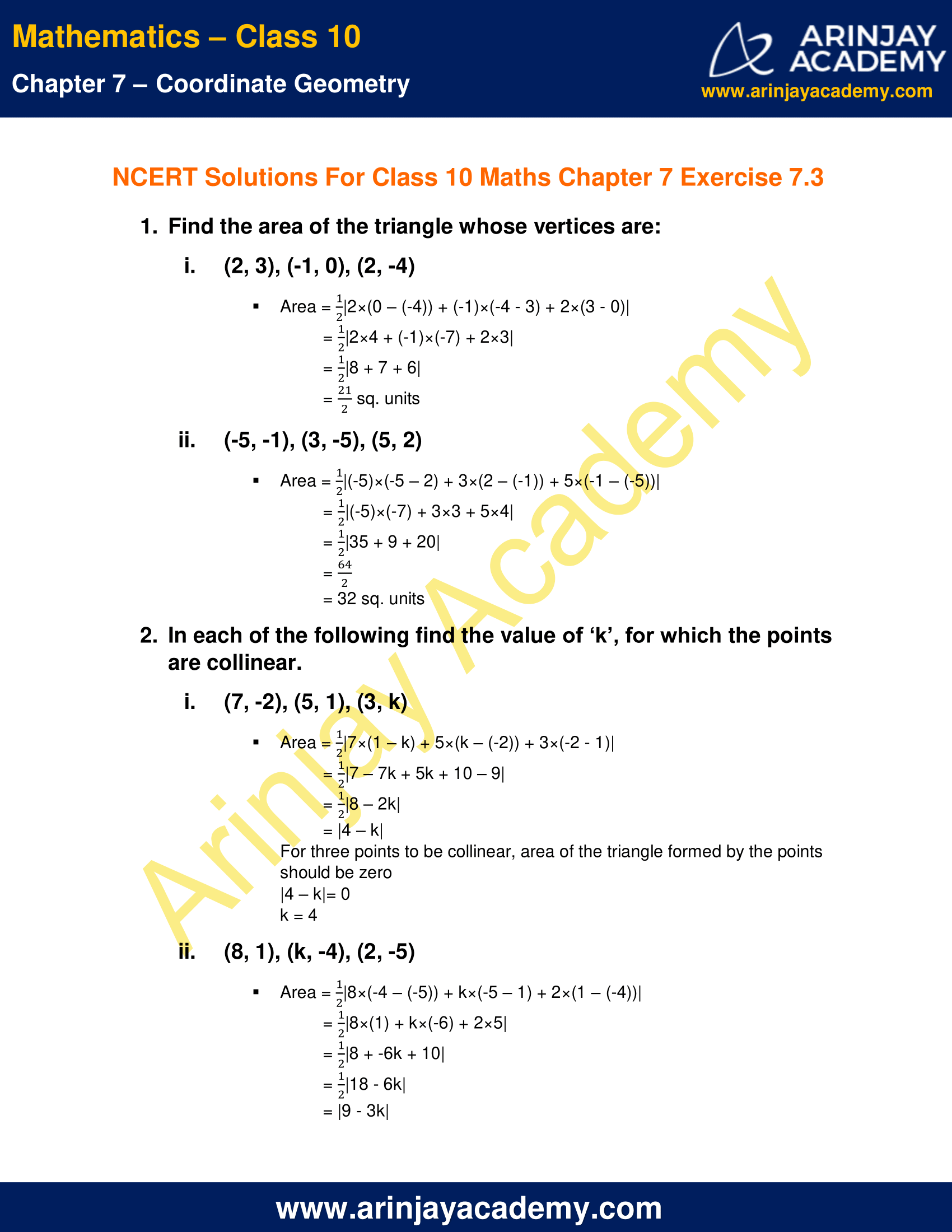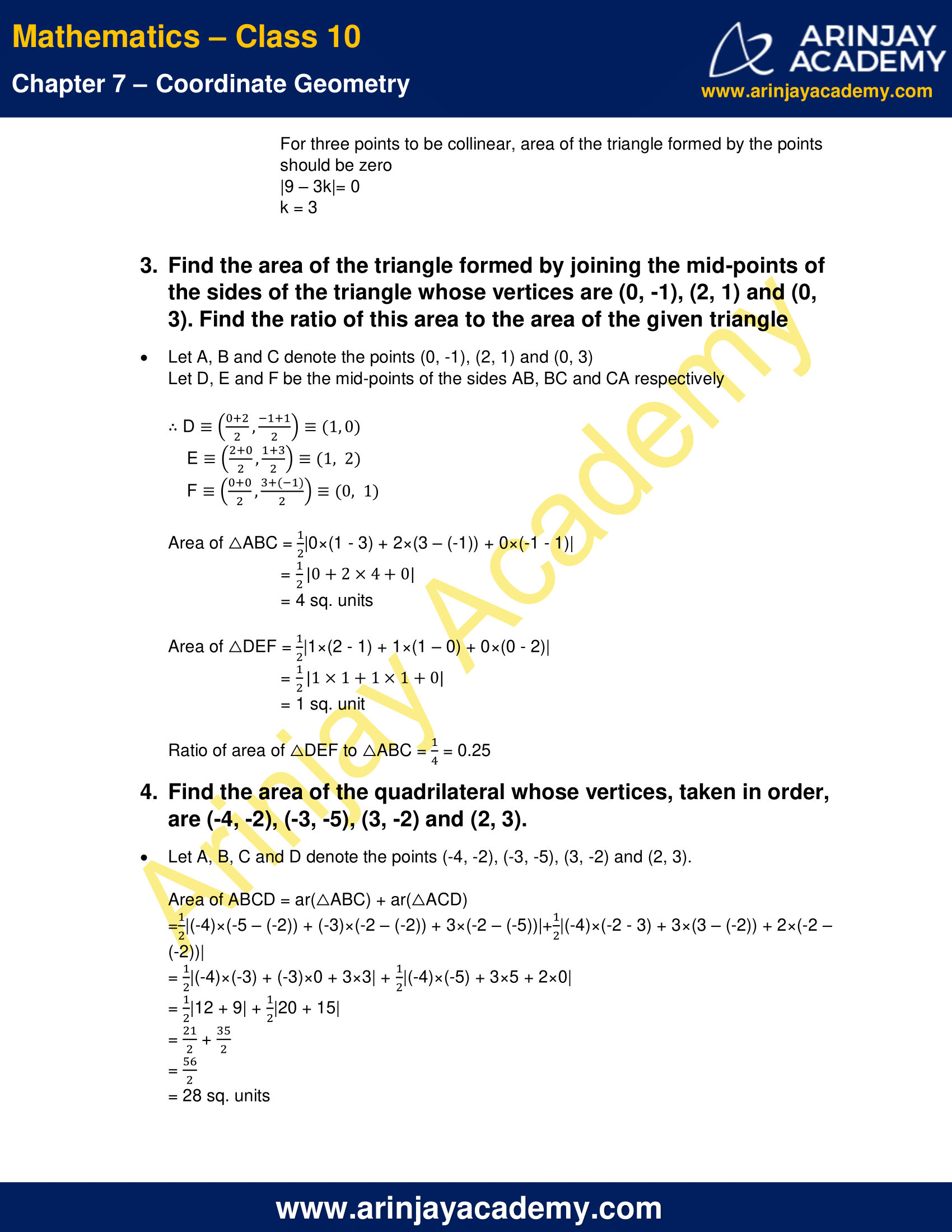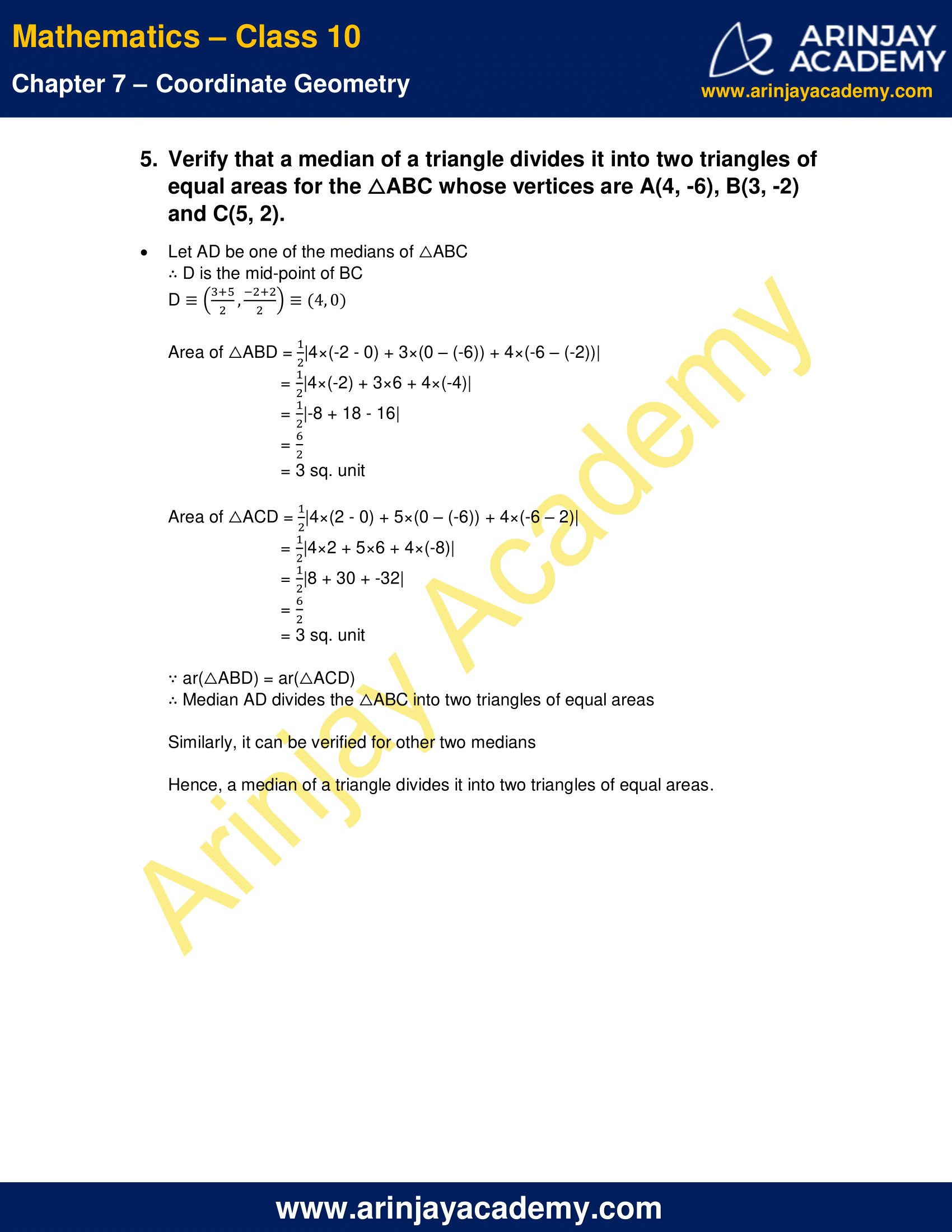# NCERT Solutions For Class 10 Maths Chapter 7 Exercise 7.3 Coordinate Geometry

Download NCERT Solutions For Class 10 Maths Chapter 7 Exercise 7.3 – Coordinate Geometry. This Exercise contains 5 questions, for which detailed answers have been provided in this note. In case you are looking at studying the remaining Exercise for Class 10 for Maths NCERT solutions for other Chapters, you can click the link at the end of this Note.

### NCERT Solutions For Class 10 Maths Chapter 7 Exercise 7.3 Coordinate GeometryNCERT Solutions For Class 10 Maths Chapter 7 Exercise 7.3 Coordinate Geometry

1. Find the area of the triangle whose vertices are:

i. (2, 3), (-1, 0), (2, -4)

Area =$\cfrac { 1 }{ 2 }$|2×(0 – (-4)) + (-1)×(-4 – 3) + 2×(3 – 0)|
=$\cfrac { 1 }{ 2 }$|2×4 + (-1)×(-7) + 2×3|
=$\cfrac { 1 }{ 2 }$|8 + 7 + 6|
=$\cfrac { 21 }{ 2 }$sq. units

ii. (-5, -1), (3, -5), (5, 2)

Area =$\cfrac { 1 }{ 2 }$|(-5)×(-5 – 2) + 3×(2 – (-1)) + 5×(-1 – (-5))|
=$\cfrac { 1 }{ 2 }$|(-5)×(-7) + 3×3 + 5×4|
=$\cfrac { 1 }{ 2 }$|35 + 9 + 20|
=$\cfrac { 64 }{ 2 }$
= 32 sq. units

2. In each of the following find the value of ‘k’, for which the points are collinear.

i. (7, -2), (5, 1), (3, k)

Area =$\cfrac { 1 }{ 2 }$|7×(1 – k) + 5×(k – (-2)) + 3×(-2 – 1)|
=$\cfrac { 1 }{ 2 }$|7 – 7k + 5k + 10 – 9|
=$\cfrac { 1 }{ 2 }$|8 – 2k|
= |4 – k|
For three points to be collinear, area of the triangle formed by the points should be zero
|4 – k|= 0
k = 4

ii. (8, 1), (k, -4), (2, -5)

Area =$\cfrac { 1 }{ 2 }$|8×(-4 – (-5)) + k×(-5 – 1) + 2×(1 – (-4))|
=$\cfrac { 1 }{ 2 }$|8×(1) + k×(-6) + 2×5|
=$\cfrac { 1 }{ 2 }$|8 + -6k + 10|
=$\cfrac { 1 }{ 2 }$|18 – 6k|
= |9 – 3k|
For three points to be collinear, area of the triangle formed by the points should be zero
|9 – 3k|= 0
k = 3

3. Find the area of the triangle formed by joining the mid-points of the sides of the triangle whose vertices are (0, -1), (2, 1) and (0, 3). Find the ratio of this area to the area of the given triangle

Let A, B and C denote the points (0, -1), (2, 1) and (0, 3)
Let D, E and F be the mid-points of the sides AB, BC and CA respectively
∴ D =$\left( \cfrac { 0+2 }{ 2 } ,\quad \cfrac { -1+1 }{ 2 } \right)$ = (1, 0)
E =$\left( \cfrac { 2+0 }{ 2 } ,\quad \cfrac { 1+3 }{ 2 } \right)$ = (1, 2)
F =$\left( \cfrac { 0+0 }{ 2 } ,\quad \cfrac { 3+(-1) }{ 2 } \right)$ = (0, 1)
Area of △ABC =$\cfrac { 1 }{ 2 }$|0×(1 – 3) + 2×(3 – (-1)) + 0×(-1 – 1)|
=$\cfrac { 1 }{ 2 }$|0+2×4+0|
= 4 sq. units
Area of △DEF =$\cfrac { 1 }{ 2 }$|1×(2 – 1) + 1×(1 – 0) + 0×(0 – 2)|
=$\cfrac { 1 }{ 2 }$|1×1+1×1+0|
= 1 sq. unit
Ratio of area of △DEF to △ABC =$\cfrac { 1 }{ 4 }$ = 0.25

4. Find the area of the quadrilateral whose vertices, taken in order, are (-4, -2), (-3, -5), (3, -2) and (2, 3).

Let A, B, C and D denote the points (-4, -2), (-3, -5), (3, -2) and (2, 3).
Area of ABCD = ar(△ABC) + ar(△ACD)
=$\cfrac { 1 }{ 2 }$|(-4)×(-5 – (-2)) + (-3)×(-2 – (-2)) + 3×(-2 – (-5))|+$\cfrac { 1 }{ 2 }$|(-4)×(-2 – 3) + 3×(3 – (-2)) + 2×(-2 – (-2))|
=$\cfrac { 1 }{ 2 }$|(-4)×(-3) + (-3)×0 + 3×3| +$\cfrac { 1 }{ 2 }$|(-4)×(-5) + 3×5 + 2×0|
=$\cfrac { 1 }{ 2 }$|12 + 9| +$\cfrac { 1 }{ 2 }$|20 + 15|
=$\cfrac { 21 }{ 2 }$ +$\cfrac { 35 }{ 2 }$
=$\cfrac { 56 }{ 2 }$
= 28 sq. units

5. Verify that a median of a triangle divides it into two triangles of equal areas for the △ABC whose vertices are A(4, -6), B(3, -2) and C(5, 2).

Let AD be one of the medians of △ABC
∴ D is the mid-point of BC
D =$\left( \cfrac { 3+5 }{ 2 } ,\quad \cfrac { -2+2 }{ 2 } \right)$ = (4, 0)
Area of △ABD =$\cfrac { 1 }{ 2 }$|4×(-2 – 0) + 3×(0 – (-6)) + 4×(-6 – (-2))|
=$\cfrac { 1 }{ 2 }$|4×(-2) + 3×6 + 4×(-4)|
=$\cfrac { 1 }{ 2 }$|-8 + 18 – 16|
=$\cfrac { 6 }{ 2 }$
= 3 sq. unit
Area of △ACD =$\cfrac { 1 }{ 2 }$|4×(2 – 0) + 5×(0 – (-6)) + 4×(-6 – 2)|
=$\cfrac { 1 }{ 2 }$|4×2 + 5×6 + 4×(-8)|
=$\cfrac { 1 }{ 2 }$|8 + 30 + -32|
=$\cfrac { 6 }{ 2 }$
= 3 sq. unit
∵ ar(△ABD) = ar(△ACD)
∴ Median AD divides the △ABC into two triangles of equal areas
Similarly, it can be verified for other two medians
Hence, a median of a triangle divides it into two triangles of equal areas.

NCERT Solutions for Class 10 Maths Chapter 7 Exercise 7.3 – Coordinate Geometry, has been designed by the NCERT to test the knowledge of the student on the topic – Area of a Triangle

Download NCERT Solutions For Class 10 Maths Chapter 7 Exercise 7.3 – Coordinate Geometry